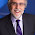## Monday, August 1, 2016

### Bearing Design Guide: Chapter One: DESIGNING THE SLEEVE BEARING

Just another day at the office…

Ring, ring….
Super Awesome Atlas Employee: Good Morning Atlas Bronze, how may I help you?
Customer:  Well…I need a bearing and I’m not sure what kind.  This is for a new project and we       are starting from scratch so I could really use some help.

HELP…did you hear that?…HELP!  Dun da na na (trumpet sound)…We have a guide for that!

Bearing Design Guide: Chapter One:  DESIGNING THE SLEEVE BEARING

First, to get started there are key things needed to know and all of these will influence the final design, material or alloy of choice.  They are as follows:

•        Speed

•       Shaft Material

•       Finishes

•       Hardness

•       Clearances

•       Lubrication

All of these factors must be considered to determine which is the most important so that the bearing can be designed to meet the highest need for the application.

The more obvious factors which are speed or type of motion, for example, rotating, oscillating or reciprocating combined with the type of load as intermittent, continuous, with or without shock or impact loading, usually point to the mode of lubrication that may be indicated.

That is, the “Pressure Velocity” factor (PV) gives a good clue of the possible success based on its product.

In order to get the PV, you need to convert the total weight (W) to be supported in pounds to the unit load (P) in pounds per square inch (PSI) and the speed in revolutions per minute to the velocity (V) in feet per minute.

Below you will fine the formulas used for Rotary Motion, Linear Motion & Oscillating Motion.

Rotary Motion

Pressure (P) = Total Load (W) I Projected Bearing Bronze Area = PSI

Note: P is the unit load in PSI

W is the total load in  pounds

PBA is the bearing diameter times the length

Velocity (V) = π x D x N/12=.262 DxN=fpm

Note: V is surface feet per minute

π is a constant 3.1416

D is the shaft or bearing dia.

N is the RPM

Liner Motion

Pressure (P) = Total Load (W) I Projected Bearing Bronze Area = PSI

Note: P is the unit load in PSI

W is the total load in pounds

PBA is the bearing diameter times the length

Velocity (V) = feet traveled per minute  (length of stroke)

Oscillating Motion

Pressure (P) = Total Load (W) I Projected Bearing Bronze Area = PSI

Note: P is the unit load in PSI

W is the total load in pounds

PBA is the bearing diameter times the length

Velocity (V) = .0029 x ID x Degree in one stroke

For applications having ideal lubrication, the PV factor
can range well above 150,000.
For severe service, the PV factor should be reduced to 90,000 .
For extreme severe duty, the PV factor should be reduced to 60,000 .

Using an arbitrary limiting bearing temperature of 250 degrees F, and using the power formula. MDWN, with an assumed constant friction factor (M) of .075, a relatively straight line value can be obtained. This result  should only be used as a guide because other factors and considerations may modify and affect the final decision.

The heat so generated  by a load (P) in combination with a low velocity (V) will be substantially less than with a low load. (P) and a high velocity (V) because velocity or speed influences the temperature  more than load or pressure does.

In actual bearing service, and as a general rule, bearing temperatures should not exceed 140 degrees F to 160 degrees F or endure a rise of more than 70 degrees F For large or heavy equipment applications, a temperature rise of 55 degrees F is acceptable.

Well...that's it for today.  I hope your head isn't spinning as much as mine was the first time I read this!  Until next time my metal loving friends...

Next Up:  Week Two, Chapter 2: Lubricating Modes

1.2.